Follow

# Calculated Field Examples

This article provides some simple formula examples to begin exploring the calculated field functionality. They are:

### Simple Arithmetic

When doing simple arithmetic the two most important things to keep in mind is to ensure that you are handling NULL values correctly and converting to the correct types.

• Subtract currency fields: CONVERT(money,ISNULL(C01,0)) - CONVERT(money,ISNULL(C02,0))
• Add decimal fields:CONVERT(decimal(17,2),ISNULL(P01,0)) + CONVERT(decimal(17,2),ISNULL(P02,0)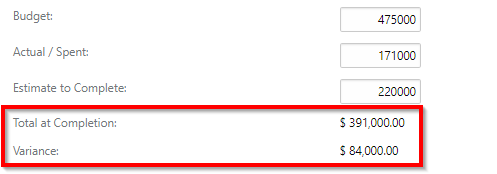### Ratios

Remember to check for division by 0 errors when calculating ratios.

• Simple ratio based on currency fields:CASE WHEN ISNULL(C02,0) = CONVERT(money,0) THEN 0 ELSE CONVERT(money,ISNULL(C04,0))  / CONVERT(money,ISNULL(C02,0)) * 100 END
• Cost variance %:CASE WHEN ISNULL(C02,0) = CONVERT(money,0) THEN 0 ELSE (CONVERT(money,ISNULL(C02,0)) - CONVERT(money,ISNULL(C04,0))) / CONVERT(money,ISNULL(C02,0)) * 100 END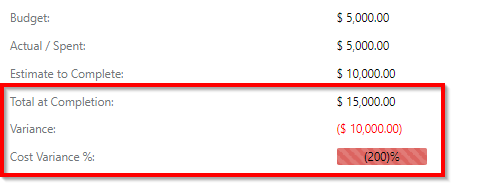### Date Calculations

• Last updated:Created
• Number of days between the date on which a record was last updated and today: DATEDIFF(day,Created, GetDate());
• Days overdue  (difference in days between issue follow-up date and current date):-(CASE WHEN I01 < GETDATE() AND IssueStatus NOT IN (466) THEN DATEDIFF(d,I01,GETDATE()) ELSE 0 END)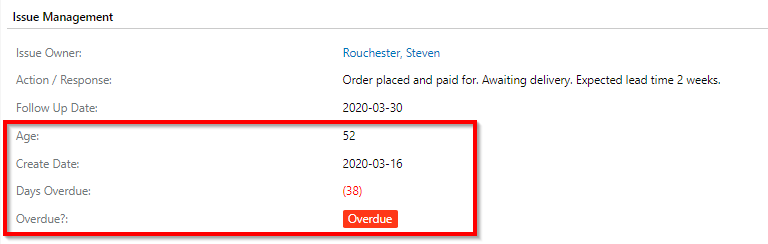### Update / Creation History

• Date on which project was created: (SELECT MIN(Created) FROM tblProjects s WHERE s.CoalescedMasterKey = tblProjects.CoalescedMasterKey)
• Captured by (the person who initially added a project): (SELECT u.EmployeeKey FROM tblUsers u WHERE u.UserKey = (SELECT TOP 1 UserKey FROM tblProjects s WHERE s.CoalescedMasterKey = tblProjects.CoalescedMasterKey ORDER BY s.Created))
• Updated by (person who last updated the record):(SELECT TOP 1 EmployeeKey FROM tblUsers u WHERE u.UserKey = tblProjects.UserKey)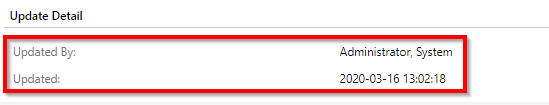### Look-Ups

• Look-up the project manager:(SELECT Manager FROM tblProjects s WHERE s.ProjectKey = IssueProjectKey)
• Look-up based on a dependency:(SELECT TOP 1 ParentKey FROM tblMappingItem WHERE MappingKey = 33 AND ChildKey = C01)
• Lookup value from a custom entity:(SELECT X05 FROM tblCustomEntity1 WHERE CE1Key = {KC01} AND MasterKey IS NULL)

### Aggregates

• Total budget for a project:CONVERT(money,ISNULL((SELECT SUM(c.CostBudget) FROM tblCost c WHERE c.CostProjectKey = tblProjects.ProjectKey AND c.MasterKey IS NULL),0))

### Users

• Check whether or not an employee is a user:  (SELECT CASE WHEN EXISTS (SELECT 1 FROM tblUsers WHERE tblEmployees.EmployeeKey = tblUsers.EmployeeKey AND tblUsers.Active = -1) THEN -1 ELSE 0 END)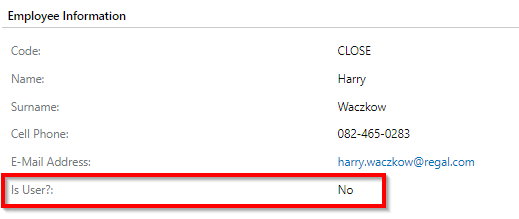### Smart Tags

You can refer to other calculated fields in your formula by placing the field's name in curly braces.

• Calculate cost variance as a percentage of the budget: CASE WHEN CostBudget = 0 THEN 0 ELSE {CostVariance} / CostBudget END
• Calculate custom list value with case statement: CASE WHEN IssueStatus IN (242) THEN 573 WHEN {IC05} < 0 THEN 570 WHEN {IC05} BETWEEN 0 AND 7 THEN 572 ELSE 571 END
• Calculate the total cost variance on a project: CONVERT(money,ISNULL((SELECT SUM({CostVariance}) FROM tblCost c WHERE c.CostProjectKey = tblProjects.ProjectKey AND c.MasterKey IS NULL),0))